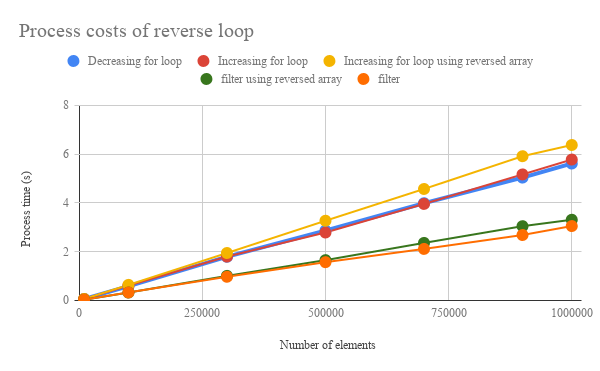Gists

Kanshi Tanaike

# Introduction

Please be careful! This result can be only used for Google Apps Script.

There are a limit executing time for Google Apps Script (GAS). That is 6 minutes. 1 So users always have to pay attention to reducing the process cost of the scripts. Especially, it is very important to know the process cost for the array processing, because the array processing is often used for spreadsheet and Google APIs. I have already reported the process costs for various processes as reports. 2-7 In this report, the process cost of “Decreasing loop” for the array processing using GAS has been investigated.

# Experimental procedure

In this report, one dimensional array was used as the sample array. The array processing was performed by “Decreasing for loop”, “Increasing for loop using reversed array” and “filter using reversed array”. About the reversed array, “Array.prototype.reverse()” was used for the created sample array. 8 The results of array processing of “Increasing for loop” and “filter” were used as the references. The process time was measured and the results were compared. In order to measure the process cost of array processing, the script which retrieves the multiple of 5 from the elements of arrays was used as a sample script. The sample scripts of GAS which were used for this report are here. a For measuring the process cost, the cost for creating array to use at the methods is not included. By the way, at GAS, the processing time is not stable as you know. So the average value for more than 100 times measurements was used for each data point which is shown by figures. At this time, the fluctuation of the average values was less than 1 %. I worry that each detailed-data point at my environment might be different from that at other user’s environment. But I think that the trend of this result can be used.

# ResultsFig 1. Number of elements vs. processing time.

Figure 1 shows number of elements vs. processing time. “Decreasing for loop”, “Increasing for loop”, “Increasing for loop using reversed array”, “filter using reversed array” and “filter” are shown as blue dots, red dots, yellow dots, green dots and orange dots, respectively. You can see each script at here. From this figure, it is found that the cost of “filter using reversed array” is lower than that of “Decreasing for loop”. For quantitatively evaluation, “filter using reversed array” makes the process cost be 43 % lower compare with “Decreasing for loop”. Also it is found that “Decreasing for loop” is almost the same with “Increasing for loop”. This is an interesting results. From “Increasing for loop using reversed array” and “Increasing for loop”, the cost of “reverse()” is 9 % of “Increasing for loop”. And from “filter using reversed array” and “filter”, the cost of “reverse()” is 6 % of “filter”. This indicates that the cost of “reverse()” is sufficiently small for each process. From these results, it was found that when the array process is run with decreasing loop, “filter using reversed array” is the best way for GAS.

# Summary

In this report, the process cost for the array processing with decreasing loop has been investigated. From this investigation, the following results were obtained.

1. “filter using reversed array” makes the process cost be 43 % lower compare with “Decreasing for loop”.
• “reversed array” was obtained using “Array.prototype.reverse()” for the created a sample array.
2. “Decreasing for loop” is almost the same with “Increasing for loop”.
3. Cost of “reverse()” is sufficiently small for “for loop” and “filter”.

As a note, I have to describe that this is the result for Google Apps Script. For other languages, this result might be difference. And also, the process cost of this report might be modified by future update of Google.

# Appendix

## Scripts

### Creating sample arrays

The function for creating array is as follows.

function make1dArray(row) {
var ar = [];
for (var i = 0; i < row; i++) {
ar[i] = i + 1;
}
return ar;
}


### For 1 dimensional array

// Decreasing for loop
var result = [];
for (var i = array.length - 1; i >= 0; i--) {
if (array[i] % 5 == 0) {
result.push(array[i]);
}
}

// Increasing for loop using reversed array
array = array.reverse();
var result = [];
for (var i = 0; i < array.length; i++) {
if (array[i] % 5 == 0) {
result.push(array[i]);
}
}

// filter using reversed array
var result = array.reverse().filter(function(e) {return e % 5 == 0});

// Increasing for loop
var result = [];
for (var i = 0; i < array.length; i++) {
if (array[i] % 5 == 0) {
result.push(array[i]);
}
}

// filter
var result = array.filter(function(e) {return e % 5 == 0});